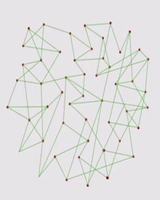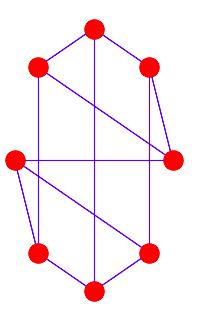GeeksforGeeks App
Open AppBrowser
Continue

# Introduction to Social Networks using NetworkX in Python

Prerequisite – Python Basics

Ever wondered how the most popular social networking site Facebook works? How we are connected with friends using just Facebook? So, Facebook and other social networking sites work on a methodology called social networks. Social networking is used in mostly all social media sites such as Facebook, Instagram, and LinkedIn, etc. It has a significant effect on marketers to engage customers. Social networks use graphs for creating a network. Their nodes are people and edges are their connection between each other. Two nodes with edges connected are friends. Now let’s see an example for understanding what is social networks.

A social network is a collection of individuals (or “nodes”) and the relationships (or “edges”) between them. Social network analysis is the process of analyzing these networks to understand patterns of interaction and communication among individuals.

NetworkX is a popular Python library for working with graphs and networks. It provides a wide range of graph algorithms and functions for creating, manipulating, and analyzing networks.

The network of 50 students in a classThe network of 50 people

The most important python library used in social networking is Networkx.

## NetworkX

NetworkX is a graph package that is used to create and modify different types of graphs. It provides a rapid development environment for collaborative, multidisciplinary projects.

#### Installation:

`pip install networkx`

After starting python, we have to import networkx module:

`import networkx as nx`

#### Basic inbuilt graph types are:

• Graph: This type of graph stores nodes and edges and edges are un-directed. It can have self-loops but cannot have parallel edges.
• Di-Graph: This type of graph is the base class for directed graphs. It can have nodes and edges and edges are directed in nature. It can have self-loops but parallel edges are not allowed in Di-Graph.
• Multi-Graph: This type of graph is an undirected graph class that can store multi or parallel edges. It can have self-loops as well. Multi-edges are multiple edges between 2 nodes.
• Multi-DiGraph: This type of graph is a directed graph class that can store multi edges. It can have self-loops as well. Multi-edges are multiple edges between 2 nodes.

## Python3

 `# import networkx library``import` `networkx as nx` `# create an empty undirected graph``G ``=` `nx.Graph()` `# adding edge in graph G``G.add_edge(``1``, ``2``)``G.add_edge(``2``, ``3``, weight``=``0.9``)`

#### Drawing of graph:

Drawing can be done using  Matplotlib.pyplot library.

## Python3

 `# import matplotlib.pyplot library``import` `matplotlib.pyplot as plt` `# import networkx library``import` `networkx as nx` `# create a cubical empty graph``G ``=` `nx.cubical_graph()` `# plotting the graph``plt.subplot(``122``)` `# draw a graph with red``# node and value edge color``nx.draw(G, pos ``=` `nx.circular_layout(G),``        ``node_color ``=` `'r'``,``        ``edge_color ``=` `'b'``)`

Output:Circular Graph

#### Graph Edge Removal:

To remove an edge from the graph, use the remove_edge() method of graph object.

Syntax: G.remove_edge(u, v)

Parameters:

• u: first node
• v: second node

Return: None

Graph Node Removal:

To remove a node from the graph, use the remove_node() method of graph object.

Syntax: G.remove_node(u)

Parameter: Node to remove

Return: None

## Python3

 `# import networkx library``import` `netwokx as nx` `# create an empty undirected graph``G ``=` `nx.Graph()` `# add edge to the graph``G.add_edge(``'1'``, ``'2'``)``G.add_edge(``'2'``, ``'3'``)` `# print the adjacent vertices``print``(G.adj)`

Output:

`{'1': {'2': {}}, '2': {'1': {}, '3': {}}, '3': {'2': {}}}`

### Advantages of using NetworkX for social network analysis include:

• A wide range of built-in algorithms: NetworkX provides a wide range of algorithms for analyzing and manipulating graphs, which makes it a powerful tool for social network analysis.
• Easy to use: NetworkX has a simple and intuitive API, which makes it easy to use even for beginners.
• Flexibility: NetworkX is built on top of the popular Python programming language, which means that it can be easily integrated with other Python libraries, such as NumPy and Matplotlib, making it a flexible tool for data analysis.
• Support for large graphs: NetworkX is built for handling large scale network analysis and visualization, it can handle large graphs with millions of nodes and edges.

### Disadvantages of using NetworkX for social network analysis include:

• Limited visualization capabilities: While NetworkX provides basic visualization capabilities, it is not as advanced as other libraries such as Gephi, which is more specialized in graph visualization.
• Limited scalability: NetworkX is not designed to handle extremely large scale graph analysis, for that other libraries such as igraph, boost.python and SNAP can be used.
• Limited support for directed and weighted edges: NetworkX is primarily designed for undirected, unweighted graphs, although it does support directed and weighted edges.

### Reference :

“Python NetworkX: A Practical Overview” by Shai Vaingast is a good reference book for learning NetworkX and its application in social network analysis. The book covers the basics of NetworkX and its use in solving real-world problems such as community detection, centrality measures, and graph visualization.

My Personal Notes arrow_drop_up